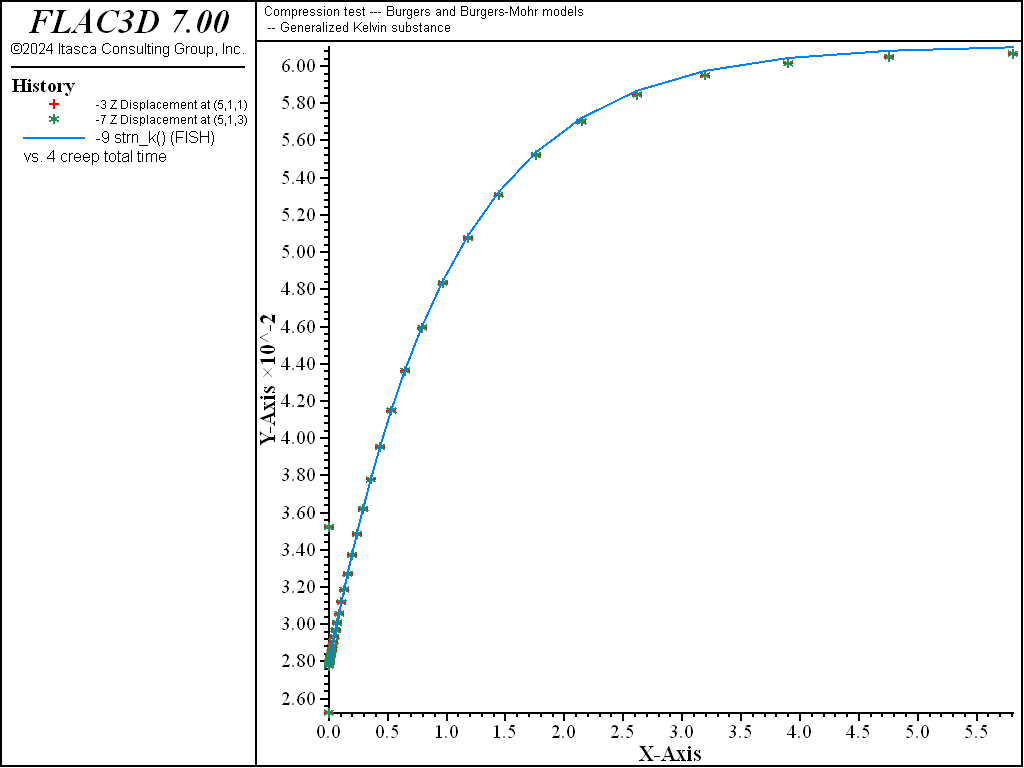# Maxwell/Burgers Model: Compression Test

Note

To view this project in FLAC3D, use the menu command Help ► Examples…. Choose “Creep/CompressionClassical” and select “CompressionClassical.prj” to load. The project’s main data files are shown at the end of this example.

This example compares numerical and analytical solutions of an unconfined compression test carried out on a Maxwell, generalized Kelvin, and Burgers substance. A Burgers substance ignoring the Maxwell viscosity will be the generalized Kelvin substance. The axial strain solutions for a constant load, $$P$$, are

(1)$\epsilon_{zz}^M = -{{P} \over {3}} \left( {t \over {\eta^M}} + {1 \over {3K}} + {1 \over {G^M}} \right)$
(2)$\epsilon_{zz}^K = -{{P} \over {3}} \left[ {1 \over {G^K}} \left( 1 - e^{-\alpha t} \right) + {1 \over {3K}} + {1 \over {G^M}} \right]$
(3)$\epsilon_{zz}^B = -{{P} \over {3}} \left[ {1 \over {G^K}} \left( 1 - e^{-\alpha t} \right) + {t \over {\eta^M}} + {1 \over {3K}} + {1 \over {G^M}} \right]$

for the Maxwell, generalized Kelvin, and Burgers substances, respectively. In those formulae, $$K$$ is bulk modulus, $$G$$ is shear modulus, $$\eta$$ is viscosity, and $$\alpha = G^K / \eta^K$$.

The numerical simulation is carried out on six samples, represented individually by one zone. Each substance is modeled using both Burgers model and Burgers-Mohr model. The cohesion property is set to a high value to prevent triggering of the plasticity logic for the Burgers-Mohr model. To model a Maxwell (or generalized Kelvin) substance, the Kelvin (or Maxwell) viscous property in the model is simply ignored. Once the elastic response is obtained (short time response), the velocities are reset to zero before proceeding to the viscous part of the simulation.

The plots of axial strain versus time, Figure 1 to Figure 3, show an instantaneous elastic response, followed by the substance characteristic creep response to a constant applied load: the creep rate is constant for the Maxwell substance, it is decreasing exponentially for the generalized Kelvin substance, and shows both transient and steady-state creep for the Kelvin substance.Figure 2: Vertical strain versus time—generalized Kelvin substance.

Data File

;------------------------------------------------------------
; Compression test -- Burgers and Burgers-Mohr models
;-------------------------------------------------------------------
model new
model large-strain off
fish automatic-create off
model title "Compression test --- Burgers and Burgers-Mohr models"
model configure creep
;
zone create brick size 5 1 3
program call 'parameter'
[setup]
zone cmodel burgers      range id-list 1 3 5
zone cmodel burgers-mohr range id-list 11 13 15
zone cmodel assign null         range id-list 2 4 6 7 8 9 10 12 14
zone property cohesion 1e20 tension 1e20 range id-list 11 13 15
;
zone face apply velocity-z 0 range union position-z 0 position-z 2
zone face apply stress-zz [P] range union position-z 1 position-z 3
;
zone history displacement-z position 1,1,1
zone history displacement-z position 3,1,1
zone history displacement-z position 5,1,1
model history creep time-total
zone history displacement-z position 1 1 3
zone history displacement-z position 3 1 3
zone history displacement-z position 5 1 3
fish history strn_m
fish history strn_k
fish history strn_b
; Burgers substance
zone property bulk [bu] shear-maxwell [g_m] viscosity-maxwell [vis_m] ...
shear-kelvin [g_k] viscosity-kelvin [vis_k] range id-list 1 11
; Maxwell substance
zone property bulk [bu] shear-maxwell [g_m] viscosity-maxwell [vis_m]  ...
range id-list 3 13
; Generalized Kelvin substance
zone property bulk [bu] shear-maxwell [g_m] shear-kelvin [g_k] ...
viscosity-kelvin [vis_k] range id-list 5 15
; --- initial "instantaneous" equilibrium ---
model solve
; --- reset velocities ---
zone gridpoint initialize velocity (0,0,0)
; --- creep test ---
model creep timestep starting 1e-5
model creep timestep automatic
model creep timestep minimum 1e-5
model creep timestep maximum 0.1
model solve time-total 6.
model save 'compression'